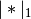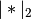# statsmodels.regression.linear_model.WLS.fit_regularized¶

`WLS.``fit_regularized`(method='coord_descent', maxiter=1000, alpha=0.0, L1_wt=1.0, start_params=None, cnvrg_tol=1e-08, zero_tol=1e-08, **kwargs)

Return a regularized fit to a linear regression model.

Parameters: method : string Only the coordinate descent algorithm is implemented. maxiter : integer The maximum number of iteration cycles (an iteration cycle involves running coordinate descent on all variables). alpha : scalar or array-like The penalty weight. If a scalar, the same penalty weight applies to all variables in the model. If a vector, it must have the same length as params, and contains a penalty weight for each coefficient. L1_wt : scalar The fraction of the penalty given to the L1 penalty term. Must be between 0 and 1 (inclusive). If 0, the fit is ridge regression. If 1, the fit is the lasso. start_params : array-like Starting values for `params`. cnvrg_tol : scalar If `params` changes by less than this amount (in sup-norm) in once iteration cycle, the algorithm terminates with convergence. zero_tol : scalar Any estimated coefficient smaller than this value is replaced with zero. A RegressionResults object, of the same type returned by `fit`.

Notes

The approach closely follows that implemented in the glmnet package in R. The penalty is the “elastic net” penalty, which is a convex combination of L1 and L2 penalties.

The function that is minimized is: ..math:

```0.5*RSS/n + alpha*((1-L1_wt)*|params|_2^2/2 + L1_wt*|params|_1)
```

where RSS is the usual regression sum of squares, n is the sample size, andandare the L1 and L2 norms.

Post-estimation results are based on the same data used to select variables, hence may be subject to overfitting biases.

References

Friedman, Hastie, Tibshirani (2008). Regularization paths for generalized linear models via coordinate descent. Journal of Statistical Software 33(1), 1-22 Feb 2010.